# ISEE Lower Level Quantitative : How to subtract

## Example Questions

1 2 3 4 6 Next →

### Example Question #392 : Isee Lower Level (Grades 5 6) Quantitative Reasoning

Joe hastotal math problems to complete for homework this week. He wants to getdone today. How many will he have left after today?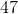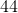Explanation:

This is a subtraction problem because we want to know how many homework problems Joe will have left after he completes some of them. We take the total number of problems that he has and subtract the number that he plans to do tonight.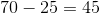### Example Question #393 : Isee Lower Level (Grades 5 6) Quantitative Reasoning

Lisa hasbirthday parties to go to this year. She has already gone toof them. How many parties does she have left to go to this year?Explanation:

This is a subtraction problem because we want to know how many birthday parties Lisa has left to attend this year. We take the total number of parties that she is invited to, and subtract the number of parties that she has already been to.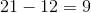### Example Question #394 : Isee Lower Level (Grades 5 6) Quantitative Reasoning

A large pizza hasslices. If we eatslices, how many will be left? (9)Explanation:

This is a subtraction problem because we want to know how many slices of pizza are left over after we have eaten some of the slices. We start with the number of silces that we started with, and subtract the number of slices that we have eaten.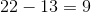.

### Example Question #49 : Use Addition And Subtraction Within 100 To Solve One And Two Step Word Problems: Ccss.Math.Content.2.Oa.A.1

Mr. Bell hasdesks in his classroom, but he only needsdesks because he hasstudents. How many extra desks does he have?Explanation:

This is a subtraction problem because we want to know how many desks are left over after all of Mr. Bell’s class sits at a desk. We take the number of desks in his room, and subtract the number of students that he has.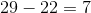### Example Question #50 : Use Addition And Subtraction Within 100 To Solve One And Two Step Word Problems: Ccss.Math.Content.2.Oa.A.1

The pet store hasgoldfish in a tank. A teacher boughtgoldfish for her school’s fish tank. How many goldfish does the pet store have left?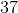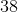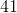This is a subtraction problem because we want to know how many goldfish the pet store has left over after the teacher buys the first and takes them away from the store. We take the number of goldfish that the store had and then subtract the number that the teacher bought.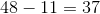.